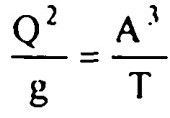top of pageSearch

# Fluid Mechanics (121-140)

Updated: Apr 21, 2020

121. For laminar flow in circular pipes, the Darcy's friction factor f is equal to

64/Rwhere R is Reynolds number

122. Surge wave in a rectangular channel is an example of

123. The best hydraulic channel cross-section is the one which has a minimum wetted perimeter

124. Which is the best hydraulic section of the following open channel cross-sections ? semi-circle

125. Hydraulic jump is a steady flow,non-uniform flow

126. The hydraulic jump always occurs from below critical depth to above critical

depth

127. In a gradually varied flow the slope of energy grade line, hydraulic grade line and bottom of channel are all different

128. The flow in channels is considered to be in transitional state if the Reynolds

number is between 500 and 2000

129. The Froude number is defined aswhere v is the mean velocity of flow, g is acceleration due to gravity and D is

hydraulic depth

130. For shooting flow the Froude number is greater than one

131. For uniform flow in a channel the total energy line, hydraulic gradient line and bottom of channel arc all parallel

132. The Chezy's coefficient has the dimension L¹/² / 2T⁻¹

133. If T is the friction factor, then the Chezy's coefficient is proportional to

134.The relationship between Manning's coefficient n and Chezy's coefficient c is

given by135.The depth of flow for maximum velocity in a circular channel section with diameter equal to 1.5 m is 1.215 m

136.For maximum discharge in a circular channel section, the ratio of the depth of

flow to that of diameter of the channel is 0.95

137.A triangular channel section is most economical when each of its sloping sides

is inclined to the vertical at an angle of 45°

138.For a trapezoidal channel section to be most economical, its hydraulic radius must be equal to y/2

139. The critical state of flow through a channel section may be defined as the

state of flow at which the discharge is maximum for a given specific force

140. The critical state of flow in a non rectangular channel is expressed by